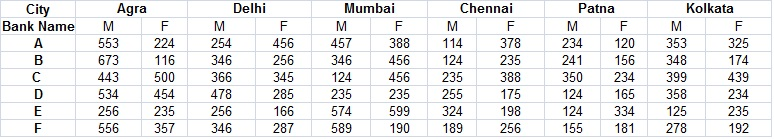## IBPS Quant Test 13

InstructionsStudy the following table carefully and answer the question that follows -
Number of employees from six different banks located in different cities M=Males,F=Females.
Table to be filled

Question 1

What is the ratio of the number of males to the number of females respectively in Patna from Bank A, Bank C and Bank E together ?

Question 2

What is the ratio of male to the number of females respectively in Bank D from all the cities together ?

Question 3

The number of females in all the banks together in Delhi are approximately what percent of the number of males from all the banks together in the same city ?

Question 4

The number of females in Bank B from Agra are what per cent of the females in bank C from the same city ?

Question 5

What is the approximate average of the numbers of males working in all the banks together in kolkata ?